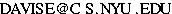# Mathematical Techniques for Computer Science Applications

G22.1180
Thursday 5:00-7:00.
Warren Weaver Hall room 312.
Professor Ernest Davis

### Reaching Me

• Email:• phone: (212) 998-3123
• office: 329 Warren Weaver Hall
Office hours: Tue 10:00-12:00, Wed 3:00-4:00

### Textbooks:

Required:
Amos Gilat, MATLAB: An Introduction with Applications, Wiley, 2008. Any edition is OK. Inexpensive used copies are available online.

Online documentation for MATLAB: Getting Started with MATLAB

### Class email list

Be sure to subscribe to the class email list

None.

### Class Notes

If you want the password before the start of class, email me.

### Description

This course gives an introduction to theory, computational techniques, and applications of linear algebra, probability and statistics. These three areas of continuous mathematics are critical in many parts of computer science, including machine learning, scientific computing, computer vision, computational biology, computational finance, natural language processing, and computer graphics. The course will teach a specialized language for mathematical computation, such as MATLAB, and will discuss how the language can be used for computation and for graphical output. No prior knowledge of linear algebra, probability, or statistics is assumed.

### Assignments

Assignment 1 due Sep. 23.
Solution to Programming Assignment 1
Exercises 1 due Sep. 23.
Solution to Exercises 1
Assignment 2 due Oct. 7.
Exercises 2 due Oct. 7.
Assignment 3 due Oct. 21.
Sample Output For Programming Assignment 3
Exercises 3 due Oct. 21.
Exercises 4 due Nov. 4.
Programming Assignment 4 due Nov. 18.
Exercises 5 due Nov. 18.
Programming Assignment 5 due Dec. 9.
Exercises 6 due Dec. 9.

### Final exam

Thursday, December 16, 5:00-7:00 PM. 312 WWH.
Study sheet

### Requirements

Biweekly programming assignments (50% of the grade).
Biweekly exercises (10% of the grade).
Final exam (40% of the grade).

### Syllabus

#### Part I. Introduction:

Week 1.A Introduction to MATLAB. Basic programming language features.
Class Notes Chapter 1: MATLAB. Gilat.

#### Part II. Linear Algebra:

Week 1.B. Vectors. Basic operations. Dot product. Vectors in MATLAB. Plotting in MATLAB.
Class Notes Chapter 2: Vectors.

Week 2. Matrices. Definition, fundamental properties, basic operations. Linear transformations.
Class Notes Chapter 3: Matrices.

Week 3. Abstract linear algebra: Linear independence, basis, rank, orthogonality, subspaces, null space.
Class Notes Chapter 4: Vector Spaces.

Week 4. Solving linear equations using Gaussian elimination.
Class Notes Chapter 5: Algorithms

Weeks 5+6. Geometric applications.
Class Notes Chapter 6: Geometry.

Week 7: Basis change and singular value decomposition.
Class Notes Chapter 7: Basis change, DFT, and SVD.

#### Part III. Probability

Week 8: Introduction. Independence. Bayes's Law. Discrete random variables.
Class Notes Chapter 8: Probability

Week 9: Numerical random variables. Expected value and variance. Discrete and continuous distributions.

Week 10: Information theory and entropy. Maximum entropy technique.

Week 11: Markov chains. Principal component analysis.

Week 12: Monte Carlo methods.

Week 13: Confidence intervals

Week 14: Flexible (overflow, review, etc.)

### Cheating

You may discuss any of the assignments with your classmates (or anyone else) but all work for all assignments must be entirely your own. Any sharing or copying of assignments will be considered cheating. By the rules of the Graduate School of Arts and Science, I am required to report any incidents of cheating to the department. Department policy is that the first incident of cheating will result in the student getting a grade of F for the course. The second incident, by GSAS rules, will result in expulsion from the University.